# The Gyroelongated Pentagonal Cupola

The gyroelongated pentagonal cupola is the 24th Johnson solid (J24). It has 25 vertices, 55 edges, and 32 faces (25 equilateral triangles, 5 squares, 1 pentagon, and 1 octagon).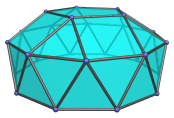The gyroelongated pentagonal cupola can be constructed by attaching a decagonal antiprism to a pentagonal cupola (J5), thereby lengthening it. The gyro in the name refers to how the bottom decagonal face is gyrated with respect to the decagonal face of the constituent pentagonal cupola.

Adding another cupola on the other side of the antiprism results in the gyroelongated pentagonal bicupola (J46). Adding a pentagonal rotunda instead produces the gyroelongated pentagonal cupolarotunda (J47).

## Projections

Here are some views of the gyroelongated pentagonal cupola from various angles:

Projection Description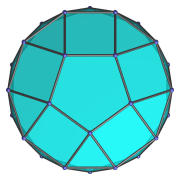Top view.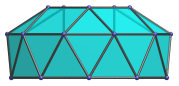Front view.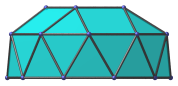Side view, with many coincident edges.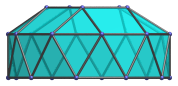9° side view, with rectangular image of antiprism.

## Coordinates

The Cartesian coordinates of the gyroelongated pentagonal cupola with edge length 2 are:

• (0, √((10+2√5)/5), 2√((3−φ)/5) + H)
• (±φ, √((5−√5)/10), 2√((3−φ)/5) + H)
• (±1, −√((5+2√5)/5), 2√((3−φ)/5) + H)
• (±2φ, 0, H)
• (±1, ±√(3+4φ), H)
• (±φ2, ±√(2+φ), H)
• (0, ±2φ, −H)
• (±√(3+4φ), ±1, −H)
• (±√(2+φ), ±φ2, −H)

where φ = (1+√5)/2 is the Golden Ratio, approximately 1.61803, and H = √(√(11φ+7)−2φ−1), approximately 0.862397.

Last updated 18 Jun 2019.